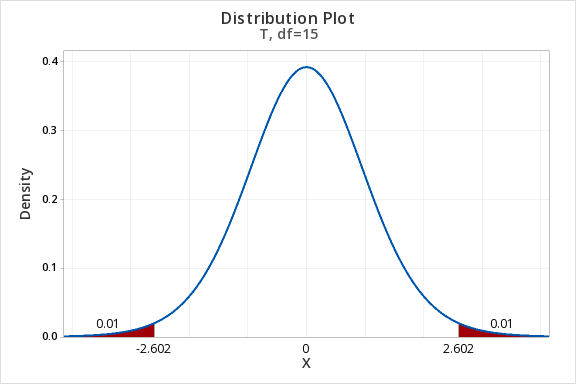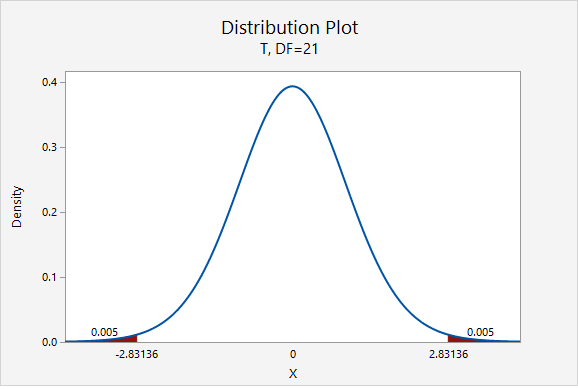# 8.2.2.1 - Formulas

8.2.2.1 - Formulas

Earlier in this lesson we considered confidence intervals for proportions and the multiplier in our intervals was a value from the standard normal (i.e., $$z$$) distribution. But, what if our variable of interest is a quantitative variable and we want to estimate a population mean?

We apply similar techniques when constructing a confidence interval for a mean, but now we are interested in estimating the population mean ($$\mu$$) by using the sample statistic ($$\overline{x}$$) and the multiplier is a $$t$$ value. Similar to the $$z$$ values that you used as the multiplier for constructing confidence intervals for population proportions, here you will use $$t$$ values as the multipliers. Because $$t$$ values vary depending on the number of degrees of freedom (df), you will need to use statistical software to look up the appropriate $$t$$ value for each confidence interval that you construct. The degrees of freedom will be based on the sample size. Since we are working with one sample here, $$df=n-1$$.

## Minitab® – Finding t* Multipliers

To find the t* multiplier for a 98% confidence interval with 15 degrees of freedom:

1. In Minitab, select Graph > Probability Distribution Plot > View Probability
2. Change the Distribution to t
3. Enter 15 for the Degrees of freedom
4. Select Options
5. Choose A specified probability
6. Select Equal tails
7. For Probability enter 0.02 (if there is 0.98 in the middle, then 0.02 is split equally between the left and right tails)

This should result in an output similar to the output below. Note that your results may be slightly different due to random sampling variation.Let’s review some of symbols and equations that we learned in previous lessons:

Sample size $$n$$ $$\mu=\frac{\sum X}{N}$$ $$\overline{x}= \frac{\sum x}{n}$$ $$SE=\frac{s}{\sqrt{n}}$$ $$t^{*}$$ $$df=n-1$$

Recall the general form for a confidence interval:

General Form of Confidence Interval
$$sample\ statistic\pm\underbrace{(multiplier)\ (standard\ error)}_{\textbf{margin of error}}$$

When constructing a confidence interval for a population mean the point estimate is the sample mean, $$\overline{x}$$. The multiplier is taken from a $$t$$ distribution. And, the standard error is equal to $$\frac{s}{\sqrt{n}}$$.

Confidence Interval for a Population Mean
$$\underbrace{\overline{x}}_{\text{sample statistic}} \pm \overbrace{t^{*}}^{\text{multiplier}} \underbrace{ \dfrac{s}{\sqrt{n}}}_{\text{standard error}}$$

On the following pages we will walk through examples of constructing confidence intervals for population means by hand. Then, you will learn how to compute confidence intervals using Minitab.

# 8.2.2.1.1 - Example: MLB Age

8.2.2.1.1 - Example: MLB Age

In a sample of 30 current MLB pitchers, the mean age was 28 years with a standard deviation of 4.4 years. Construct a 95% confidence interval to estimate the mean age of all current MLB pitchers.

This is what we know: $$n=30$$, $$\overline{x}=28$$, and $$s=4.4$$.

In order to compute the confidence interval for $$\mu$$ we will need the t multiplier and the standard error ($$\frac{s}{\sqrt{n}}$$).

$$df=n-1=30-1=29$$For a 95% confidence interval with 29 degrees of freedom, $$t^{*}=2.045$$

$$SE=\dfrac{s}{\sqrt{n}}=\dfrac{4.4}{\sqrt{30}}=0.803$$

Thus, our confidence interval for $$\mu$$ is: $$28\pm 2.045(0.803)=28\pm1.643=[26.357,29.643]$$

We are 95% confident that the population mean age is between 26.357 and 29.643.

# 8.2.2.1.2- Example: Sleep Deprivation

8.2.2.1.2- Example: Sleep Deprivation

In a class survey, students were asked how many hours they sleep per night. In the sample of 22 students, the mean was 5.77 hours with a standard deviation of 1.572 hours. That distribution was approximately normal. Let’s construct a 95% confidence interval for the mean number of hours slept per night in the population from which this sample was drawn.

This is what we know: $$n=22$$, $$\overline{x}=5.77$$, and $$s=1.572$$.

In order to compute the confidence interval for $$\mu$$ we will need the t multiplier and the standard error ($$\frac{s}{\sqrt{n}}$$).

$$df=n-1=22-1=21$$For a 95% confidence interval with 21 degrees of freedom, $$t^{*}=2.080$$

$$SE=\frac{s}{\sqrt{n}}=\frac{1.572}{\sqrt{22}}=0.335$$

Thus, our confidence interval for $$\mu$$ is: $$5.77\pm 2.080(0.335)=5.77\pm0.697=[5.073,\;6.467]$$

We are 95% confident that the population mean is between 5.073 and 6.467 hours.

What if we wanted to be more conservative and construct a 99% confidence interval?

The only thing that would change is our multiplier. Now, $$t^{*}=2.831$$.$$5.77\pm 2.831(0.335)=5.77\pm0.948=[4.822,\;6.718]$$

We are 99% confident that the population mean is between 4.822 and 6.718 hours.

# 8.2.2.1.3 - Example: Milk

8.2.2.1.3 - Example: Milk

A study of 66,831 dairy cows found that the mean milk yield was 12.5 kg per milking with a standard deviation of 4.3 kg per milking (data from Berry, et al., 2013). Construct a 95% confidence interval for the average milk yield in the population.

First, let's compute the standard error:

$$SE=\dfrac{s}{\sqrt{n}}=\dfrac{4.3}{\sqrt{66831}}=0.0166$$

The standard error is small because the sample size is very large.

Next, let's find the $$t^*$$ multiplier:

$$df=66831-1=66830$$$$t^{*}=1.960$$

Now, we can construct our 95% confidence interval:

95% C.I.: $$12.5\pm1.960(0.017)=12.5\pm0.033=[12.467,\;12.533]$$

We are 95% confident that the mean milk yield in the population is between 12.467 and 12.533 kg per milking.

  Link ↥ Has Tooltip/Popover Toggleable Visibility